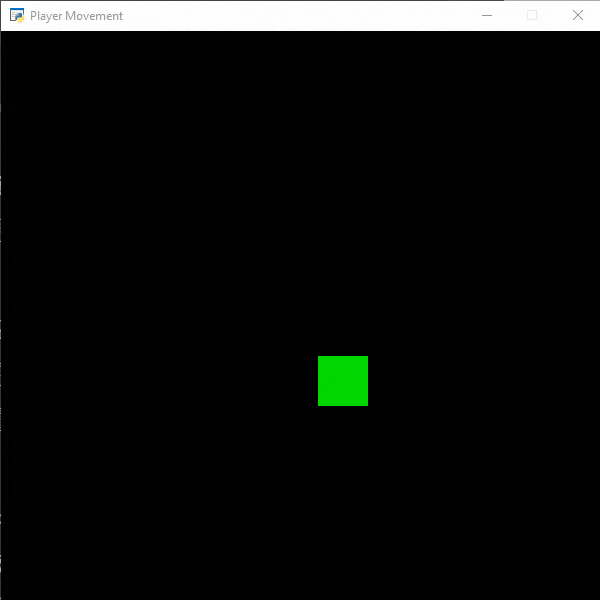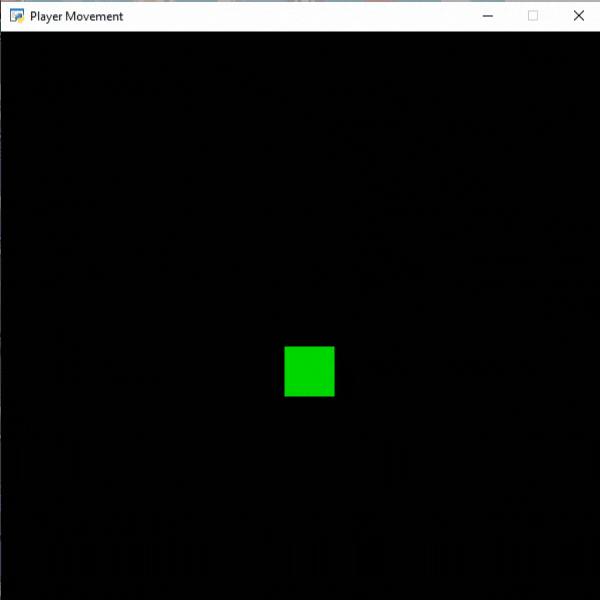# Python Arcade – Player Movement

• Difficulty Level : Hard
• Last Updated : 23 Sep, 2021

## Automatic Movements

We can easily move our players in any particular direction in the arcade. For this, we are going to draw a rectangle using the draw_rectangle_filled() method then we will change the x coordinate of this rectangle.

Attention geek! Strengthen your foundations with the Python Programming Foundation Course and learn the basics.

To begin with, your interview preparations Enhance your Data Structures concepts with the Python DS Course. And to begin with your Machine Learning Journey, join the Machine Learning - Basic Level Course

Syntax: arcade.draw_rectangle_filled(x, y, width, height, color, angle)

Parameters:

• x : x coordinate of the rectangle center
• y : y coordinate of the rectangle center
• width : width of the rectangle
• height : height of the rectangle
• color : color of the rectangle
• angle : rotation of the rectangle.

Below is the implementation:

## Python3

 `# Importing arcade module``import` `arcade`` ` `# Creating MainGame class       ``class` `MainGame(arcade.Window):``    ``def` `__init__(``self``):``        ``super``().__init__(``600``, ``600``, title``=``"Player Movement"``)`` ` `        ``# Initializing the initial x and y coordinated``        ``self``.x ``=` `250` `        ``self``.y ``=` `250`` ` `        ``# Initializing a variable to store``        ``# the velocity of the player``        ``self``.vel ``=` `300`` ` `    ``# Creating on_draw() function to draw on the screen``    ``def` `on_draw(``self``):``        ``arcade.start_render()`` ` `        ``# Drawing the rectangle using``        ``# draw_rectangle_filled function``        ``arcade.draw_rectangle_filled(``self``.x, ``self``.y,``50``, ``50``,``                                     ``arcade.color.GREEN )``    ``# Creating on_update function to``    ``# update the x coordinate``    ``def` `on_update(``self``,delta_time):``        ``self``.x ``+``=` `self``.vel ``*` `delta_time`` ` `        ``# Changing the direction of``        ``# movement if player crosses the screen``        ``if` `self``.x>``=``550` `or` `self``.x<``=``50``:``            ``self``.vel ``*``=` `-``1``         ` `# Calling MainGame class       ``MainGame()``arcade.run()`

Output:## Player Movement using Keyboard Inputs

In arcade, we can take inputs from users to move our players. For this, we will use the on_key_press() and on_key_release() function.

Syntax:

• on_key_press(symbol,modifiers)
• on_key_release) symbol, modifiers)

Parameters:

• symbol: Key that was hit
• modifiers: Bitwise ‘and’ of all modifiers (shift, ctrl, num lock) pressed during this event

Below is the implementation:

## Python3

 `# Importing arcade module``import` `arcade`` ` `# Creating MainGame class       ``class` `MainGame(arcade.Window):``    ``def` `__init__(``self``):``        ``super``().__init__(``600``, ``600``, title``=``"Player Movement"``)`` ` `        ``# Initializing the initial x and y coordinated``        ``self``.x ``=` `250` `        ``self``.y ``=` `250`` ` `        ``# Initializing a variable to store``        ``# the velocity of the player``        ``self``.vel_x ``=` `0``        ``self``.vel_y ``=` `0`` ` `    ``# Creating on_draw() function to draw on the screen``    ``def` `on_draw(``self``):``        ``arcade.start_render()`` ` `        ``# Drawing the rectangle using``        ``# draw_rectangle_filled function``        ``arcade.draw_rectangle_filled(``self``.x, ``self``.y,``50``, ``50``,``                                     ``arcade.color.GREEN )``    ``# Creating on_update function to``    ``# update the x coordinate``    ``def` `on_update(``self``,delta_time):``        ``self``.x ``+``=` `self``.vel_x ``*` `delta_time``        ``self``.y ``+``=` `self``.vel_y ``*` `delta_time`` ` `         ` `    ``# Creating function to change the velocity``    ``# when button is pressed``    ``def` `on_key_press(``self``, symbol,modifier):`` ` `        ``# Checking the button pressed``        ``# and changing the value of velocity``        ``if` `symbol ``=``=` `arcade.key.UP:``            ``self``.vel_y ``=` `300``        ``elif` `symbol ``=``=` `arcade.key.DOWN:``            ``self``.vel_y ``=` `-``300``        ``elif` `symbol ``=``=` `arcade.key.LEFT:``            ``self``.vel_x ``=` `-``300``        ``elif` `symbol ``=``=` `arcade.key.RIGHT:``            ``self``.vel_x ``=` `300`` ` `    ``# Creating function to change the velocity``    ``# when button is released``    ``def` `on_key_release(``self``, symbol, modifier):`` ` `        ``# Checking the button released``        ``# and changing the value of velocity``        ``if` `symbol ``=``=` `arcade.key.UP:``            ``self``.vel_y ``=` `0``        ``elif` `symbol ``=``=` `arcade.key.DOWN:``            ``self``.vel_y ``=` `0``        ``elif` `symbol ``=``=` `arcade.key.LEFT:``            ``self``.vel_x ``=` `0``        ``elif` `symbol ``=``=` `arcade.key.RIGHT:``            ``self``.vel_x ``=` `0``         ` `         ` `# Calling MainGame class       ``MainGame()``arcade.run()`

Output:My Personal Notes arrow_drop_up